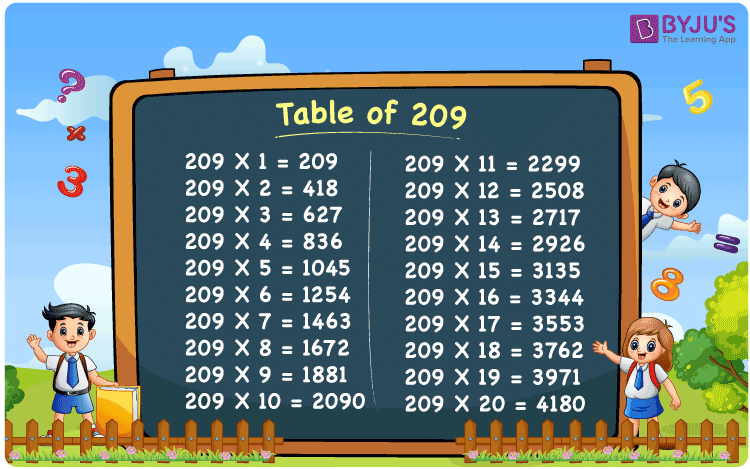Checkout JEE MAINS 2022 Question Paper Analysis : Checkout JEE MAINS 2022 Question Paper Analysis :

# Table of 209

The Table of 209 is given here up to 20 times to help students check the values of multiples of 209. Download printable PDF of the table of 209 use it for memorising the multiples of 209, which will improve mental maths skills.

## Table of 209 Chart

The table of 209 chart is presented here with an attractive image that can be downloaded to use as a flashcard for future reference. The 209 times table is essential for quick calculations which will be useful for many competitive examinations.## What is the 209 Times Table?

The 209 times table is a list of the multiples of 209 that are obtained by the multiplication of 209 with various natural numbers. The 209 times table is given here both in multiplication and addition form.

 209×1 = 209 209 209×2 = 418 209 + 209 = 418 209×3 = 627 209 + 209 + 209 = 627 209×4 = 836 209 + 209 + 209 + 209 = 836 209×5 = 1045 209 + 209 + 209 + 209 + 209 = 1045 209×6 = 1254 209 + 209 + 209 + 209 + 209 + 209 = 1254 209×7 = 1463 209 + 209 + 209 + 209 + 209 + 209 + 209 = 1463 209×8 = 1672 209 + 209 + 209 + 209 + 209 + 209 + 209 + 209 = 1672 209×9 = 1881 209 + 209 + 209 + 209 + 209 + 209 + 209 + 209 + 209 = 1881 209×10 = 2090 209 + 209 + 209 + 209 + 209 + 209 + 209 + 209 + 209 + 209 = 2090

## Multiplication Table of 209

Below given is a tabular list of the multiples of 209 up to 20.

 209 × 1 = 209 209 × 2 = 418 209 × 3 = 627 209 × 4 = 836 209 × 5 = 1045 209 × 6 = 1254 209 × 7 = 1463 209 × 8 = 1672 209 × 9 = 1881 209 × 10 = 2090 209 × 11 = 2299 209 × 12 = 2508 209 × 13 = 2717 209 × 14 = 2926 209 × 15 = 3135 209 × 16 = 3344 209 × 17 = 3553 209 × 18 = 3762 209 × 19 = 3971 209 × 20 = 4180

## Solved Examples on the Table of 209

Example 1:

What is the difference between 13 times 209 and 19 times 209?

Solution:

Using the multiplication table of 209

13 times 209 = 2717

19 times 209 = 3971

Therefore, 3971 – 2717 = 1254

Example 2:

Evaluate the half of 20 times 209.

Solution:

20 times 209 = 4180

Then, the half of 4180 = ½  × 4180 = 2090

## Frequently Asked Questions on Table of 209

### What is the times tables of 209?

The 209 times table is the multiplication of 209 with natural numbers. Starting with 209 × 1 = 209, 209 × 2 = 418, 209 × 3 = 627, 209 × 4 = 836, 209 × 5 = 1045 and so on.

### What times table has 209?

209 comes in the times tables of 1, 11, 19 and 209.

### What are the factors of 209?

The factors of 209 are 1, 11, 19 and 209. The prime factors of 209 are 11 and 19, and the prime factorisation of 209 is 11 × 19.

### Is 209 a prime number?

No, 209 is a composite number.

### Is 209 divisible by 11?

Yes, 209 is divisible by 11, 209 = 11 × 19.